Two Transistor Model of a Thyristor

# Two Transistor Model of a Thyristor - Notes | Study Power Electronics - Electrical Engineering (EE)

 1 Crore+ students have signed up on EduRev. Have you?

Basic operating principle of SCR, can easily be understood by the two transistor model of SCR, as it is a combination of p and n layers.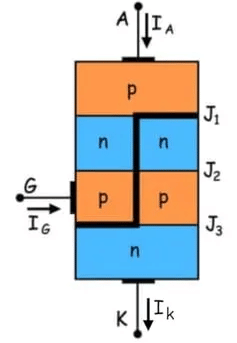This is a pnpn thyristor. If we bisect it through the dotted line then we will get two transistors i.e. one pnp transistor with J1 and J2 junctions and another is with J2 and J3 junctions as shown in figure below.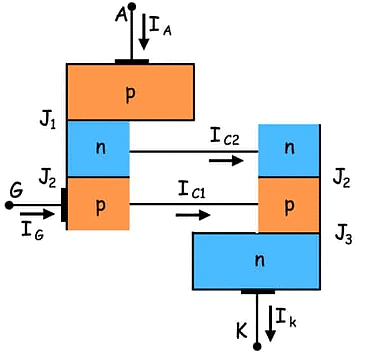The relation between the collector current and emitter current is shown below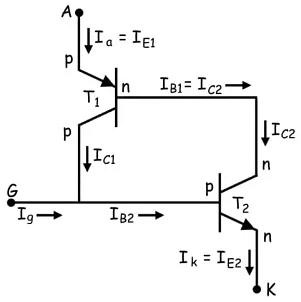Here, IC is collector current, IE is emitter current, ICBO is forward leakage current, α is common base forward current gain and relationship between IC and IB is
I= βIB

Where, IB is base current and β is common emitter forward current gain.

Let’s for transistor T1 this relation holds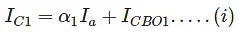And that for transistor T2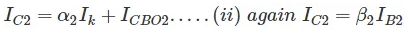Now, by the analysis of two transistors model we can get anode current,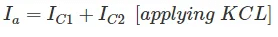From equation (i) and (ii), we get,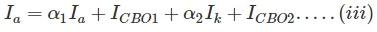If applied gate current is Ig then cathode current will be the summation of anode current and gate current i.e.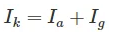By substituting this valyue of Ik in (iii) we get,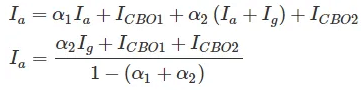From this relation we can assure that with increasing the value of
α1 + α2
towards unity, corresponding anode current will increase. Now the question is how
α1 + α2
increasing? Here is the explanation using two transistor model of SCR.
At the first stage when we apply a gate current Ig, it acts as base current of T2 transistor i.e. IB2 = Ig and emitter current of the T2 transistor IE2 = Ik. Hence establishment of the emitter current gives rise α2 as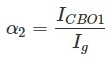Presence of base current will generate collector current as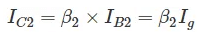This IC2 is nothing but base current IB1 of transistor T1, which will cause the flow of collector current,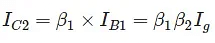IC1 and IB1 lead to increase IC1 as
and hence, α1 increases. Now, new base current of T2 is,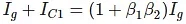which will lead to increase emitter current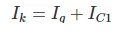and as a result α2 also increases and this further increases.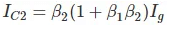As, α1 again increases. This continuous positive feedback effect increases
+ α2)
towards unity and anode current tends to flow at a very large value. The value current then can only be controlled by external resistance of the circuit.

The document Two Transistor Model of a Thyristor - Notes | Study Power Electronics - Electrical Engineering (EE) is a part of the Electrical Engineering (EE) Course Power Electronics.
All you need of Electrical Engineering (EE) at this link: Electrical Engineering (EE)

## Power Electronics

5 videos|39 docs|63 tests
 Use Code STAYHOME200 and get INR 200 additional OFF

## Power Electronics

5 videos|39 docs|63 tests

Track your progress, build streaks, highlight & save important lessons and more!

,

,

,

,

,

,

,

,

,

,

,

,

,

,

,

,

,

,

,

,

,

;